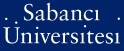# Comparison of fuzzy extent analysis technique and its extensions with original eigen vector approach

Ahmed, Faran and Kılıç, Kemal (2016) Comparison of fuzzy extent analysis technique and its extensions with original eigen vector approach. In: 18th International Conference on Enterprise Information Systems (ICEIS 2016), Rome, ItalyPDF - Registered users only - Requires a PDF viewer such as GSview, Xpdf or Adobe Acrobat Reader387Kb

## Abstract

Fuzzy set theory has been extensively incorporated in the original Analytical Hierarchical Process (AHP) with an aim to better represent human judgments in comparison matrices. One of the most popular technique in the domain of Fuzzy AHP is Fuzzy Extent Analysis method which utilizes the concept of extent analysis combined with degree of possibility to calculate weights from fuzzy comparison matrices. In original AHP, where the comparison matrices are composed of crisp numbers, Satty proposed that Eigen Vector of these comparison matrices estimate the required weights. In this research we perform a comparison analysis of these two approaches based on a data set of matrices with varying level of inconsistency. Furthermore, for the case of FEA, in addition to degree of possibility, we use centroid defuzzification and defuzzification by using the mid number of triangular fuzzy number to rank the final weights calculated from fuzzy comparison matrices.

Item Type: Papers in Conference Proceedings Eigen vector approach; Fuzzy AHP; Fuzzy Extent Analysis UNSPECIFIED 29582 Kemal Kılıç 02 Sep 2016 14:26 20 Sep 2016 10:22

Repository Staff Only: item control page